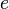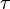## What is a PID controller?## Introduction

A PID (Proportional Integral Derivative) controller is a common instrument used in industrial control applications. A PID controller can be used for regulation of speed, temperature, flow, pressure and other process variables. Field mounted PID controllers can be placed close to the sensor or the control regulation device and be monitored centrally using a SCADA system.where: Proportional gain, a tuning parameter: Integral gain, a tuning parameter: Derivative gain, a tuning parameter: Error: Time or instantaneous time (the present): Variable of integration; takes on values from time 0 to the present.

## Description

A proportional–integral–derivative controller (PID controller) is a generic control loop feedback mechanism (controller) widely used in industrial control systems – a PID is the most commonly used feedback controller. A PID controller calculates an “error” value as the difference between a measured process variable and a desired setpoint. The controller attempts to minimize the error by adjusting the process control inputs.

The PID controller calculation (algorithm) involves three separate constant parameters, and is accordingly sometimes called three-term control: the proportional, the integral and derivative values, denoted P, I, and D. Heuristically, these values can be interpreted in terms of time: P depends on the present error, I on the accumulation of past errors, and D is a prediction of future errors, based on current rate of change.The weighted sum of these three actions is used to adjust the process via a control element such as the position of a control valve, or the power supplied to a heating element.In the absence of knowledge of the underlying process, a PID controller has historically been considered to be the best controller. By tuning the three parameters in the PID controller algorithm, the controller can provide control action designed for specific process requirements. The response of the controller can be described in terms of the responsiveness of the controller to an error, the degree to which the controller overshoots the setpoint and the degree of system oscillation. Note that the use of the PID algorithm for control does not guarantee optimal control of the system or system stability.

Some applications may require using only one or two actions to provide the appropriate system control. This is achieved by setting the other parameters to zero. A PID controller will be called a PI, PD, P or I controller in the absence of the respective control actions. PI controllers are fairly common, since derivative action is sensitive to measurement noise, whereas the absence of an integral term may prevent the system from reaching its target value due to the control action.Plot of PV vs time, for three values of Kp (Ki and Kd held constant)Plot of PV vs time, for three values of Ki (Kp and Kd held constant)Plot of PV vs time, for three values of Kd (Kp and Ki held constant)

## 7 thoughts on “What is a PID controller?”

1.prernajain26 says:

pid controller has its pros and cons

2.yashwini says:

i loved your website, it always help me when i want to understand more about some subject, thanks a lot for helping us to get this information

3.Taisha Coursey says:

nice